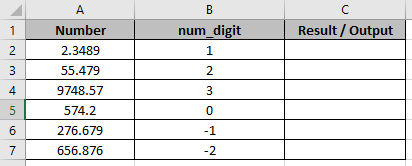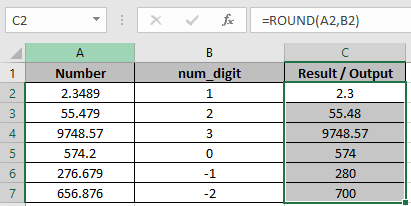# How to Use Round Function in Excel

In this article, we will learn how to use Round Function to round off the numbers up to the nearest number in Excel.
ROUND function used to round down the numbers 0-4 and round up the numbers 5-9 It takes 2 arguments

• The Number and
• Up to digit, we need to round off.

Syntax:

=ROUND(number, num_digit)

Let’s use them in some examples to see how it works.In one Column there are Numbers and in other, there are num_digits up to which the number to be rounded off.

Use the formula in C2 cell.

=ROUND(A2,B2)

Explanation:
Here in C2 cell, the number will be round down to 1 decimal place as 3 comes in 1-4.As you can see 2.3 is rounded down to 1 decimal place.
Copy the formula using Ctrl + D taking the first cell till the last cell needed formula.As you can see the result. Cell C2, C4 and C5 are rounded down and Cell C3, C6 and C7 are rounded up.

The 2nd argument(num_digit) decides upto which decimal or 10s place the number will be rounded off.

1. First 3 cells are rounded off upto 1, 2 and 3 decimal places respectively.
2. In C5, The number is rounded off to the nearest whole number.
3. The last 2 cells are rounded off to the nearest 10 and 100 respectively.
To get the number rounded off upto the nearest whole number, 0 is used default as num_digit

Hope you understood how to use ROUND function in Excel. Explore more articles on ROUNDUP and ROUNDDOWN function here. State your queries in the comment box below.

Related Articles:

Formula Round to nearest 100 and 1000

How to Use MROUND function in Excel

How to Use RoundUp function in Excel

How to Use Rounddown function in Excel

Rounding Numerical Calculation Results In Microsoft Excel

Popular Articles:

How to use the VLOOKUP Function in Excel

How to use the COUNTIF function in Excel 2016

How to Use SUMIF Function in Excel

Terms and Conditions of use

The applications/code on this site are distributed as is and without warranties or liability. In no event shall the owner of the copyrights, or the authors of the applications/code be liable for any loss of profit, any problems or any damage resulting from the use or evaluation of the applications/code.